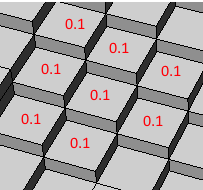# How to label model according to cell data?

I have model (*.vtu) containing several blocks like:

I want to label values on each block like:How can I do it ? I can access values using:

``````vtkActor actor = ...;
var output = actor.GetMapper().GetInputAsDataSet();
var cell = output.GetCellData();
for (int i = 0; i < cell.GetNumberOfComponents(); i++)
{
var cells = cell.GetArray(i);
if (cells == null) continue;
if ("IJK" != cell.GetArrayName(i)) continue; //There are many columns. I used IJK column as testing purpose
var min = cells.GetRange();
var max = cells.GetRange();
for (int j = 0; j < nCells; j++)
{
double val = cells.GetComponent(j, i);
// how to label this value now ?
}
break;
}
vtkDataSetMapper mapper = vtkDataSetMapper.New();
mapper.SetInput(output);
actor.SetMapper(mapper);
``````

Here’s attached my model file: model.zip (3.1 MB)